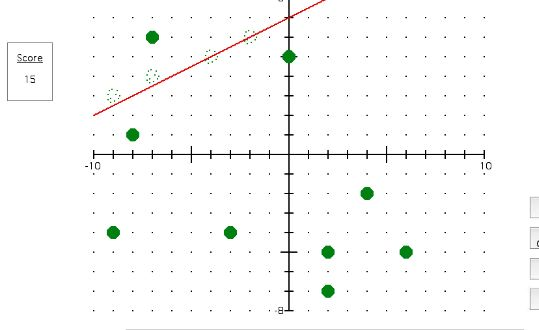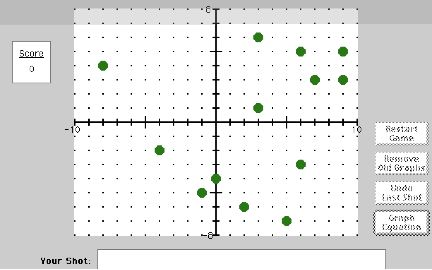The Great Green Globs Contest Worksheet 15: Instructions and Tips by Sharon Dugdale and David KibbeyGREEN GLOBS is a strategy game using graphs of equations.  Thirteen randomly scattered green globs are displayed on a coordinate grid.  Your goal is to explode all of the globs.  The globs explode when they are hit by the graph of an equation that passes through them.  You enter equations at the keyboard.

Levels of Difficulty
The Novice Game is the best place to start.  You cannot use trigonometric functions in the Novice game.  The Expert Game is more difficult.  You can use trigonometric functions; but there are five ―shot absorbers‖ displayed on the grid.  The shot absorbers stop any graphs that hit them.

Scoring
For each equation you enter, the first glob hit is worth one point, the second glob is worth two points, the third is worth four points, the fourth is worth eight points, and so on.  For example, if you hit four globs with one equation, you score is 1 + 2 + 4 + 8 = 15 points.

Equations
Try to hit as many globs as possible with each graph.  You may use equations of the following types:
•  straight lines
•  functions beginning with y =  or  x =
•  equations for conics (such as circles and hyperbolas)
•  functions using square roots or absolute value
•  equations using logarithmic or exponential functions

Trigonometric functions can be used only in the Expert Game.

Top Scores and Games
In both the Novice and Expert Games, the top ten scores and the games that produced them can be shown in the records.  After you try a few games of GREEN GLOBS, you may want to look at the records.

Copyright © 1996, 2008 by Sharon Dugdale and David Kibbey  p. 67 (User Guide)